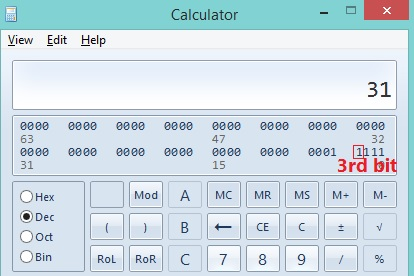# How to check a particular bit is SET or not using C program?

Learn: How to check whether a bit is SET (High) or not using C programming language? Here, we will read a number and bit and check input bit is SET or not.

Bitwise AND Operator (&) is used to check whether a bit is SET (HIGH) or not SET (LOW) in C and C++ programming language.

Bitwise AND Operator (&) is a binary operator, which operates on two operands and checks the bits, it returns 1, if both bits are SET (HIGH) else returns 0.

Let suppose, you want to check Nth bit of a Number NUM, you can do the same by following this syntax:

(NUM & (1<<N))

Here, NUM is the number whose bit you want to check and N is the bit number, (1<<N) SET the particular bit at Nth position.

Example: Statement (1<<3) returns 8 (in Binary: 0000 1000), see the binary 3rd (count from 0 to 7) bit is SET here.

In this program:

```Input:
Enter an 8 bits integer number: 31
Now, enter a bit number (from 0 to 7) to check, whether it is SET or not: 3
Output:
Bit number 3 is SET in number 31
```

## Program to check whether bit is SET or not in C language

```#include <stdio.h>

int main()
{
int NUM; //to store number
int N; //to store bit

printf("Enter an 8 bits integer number: ");
scanf("%d",&NUM);
printf("Now, enter a bit number (from 0 to 7) to check, whether it is SET or not: ");
scanf("%d",&N);

//checking bit status
if(NUM & (1<<N))
printf("Bit number %d is SET in number %d.\n",N,NUM);
else
printf("Bit number %d is not SET in number %d.\n",N,NUM);

return 0;
}
```

Output

```Enter an 8 bits integer number: 31
Now, enter a bit number (from 0 to 7) to check, whether it is SET or not: 3
Bit number 3 is SET in number 31.
```

Here, the input was 31, which is 0001 1111 and its 3rd bit is SET.

See the image: